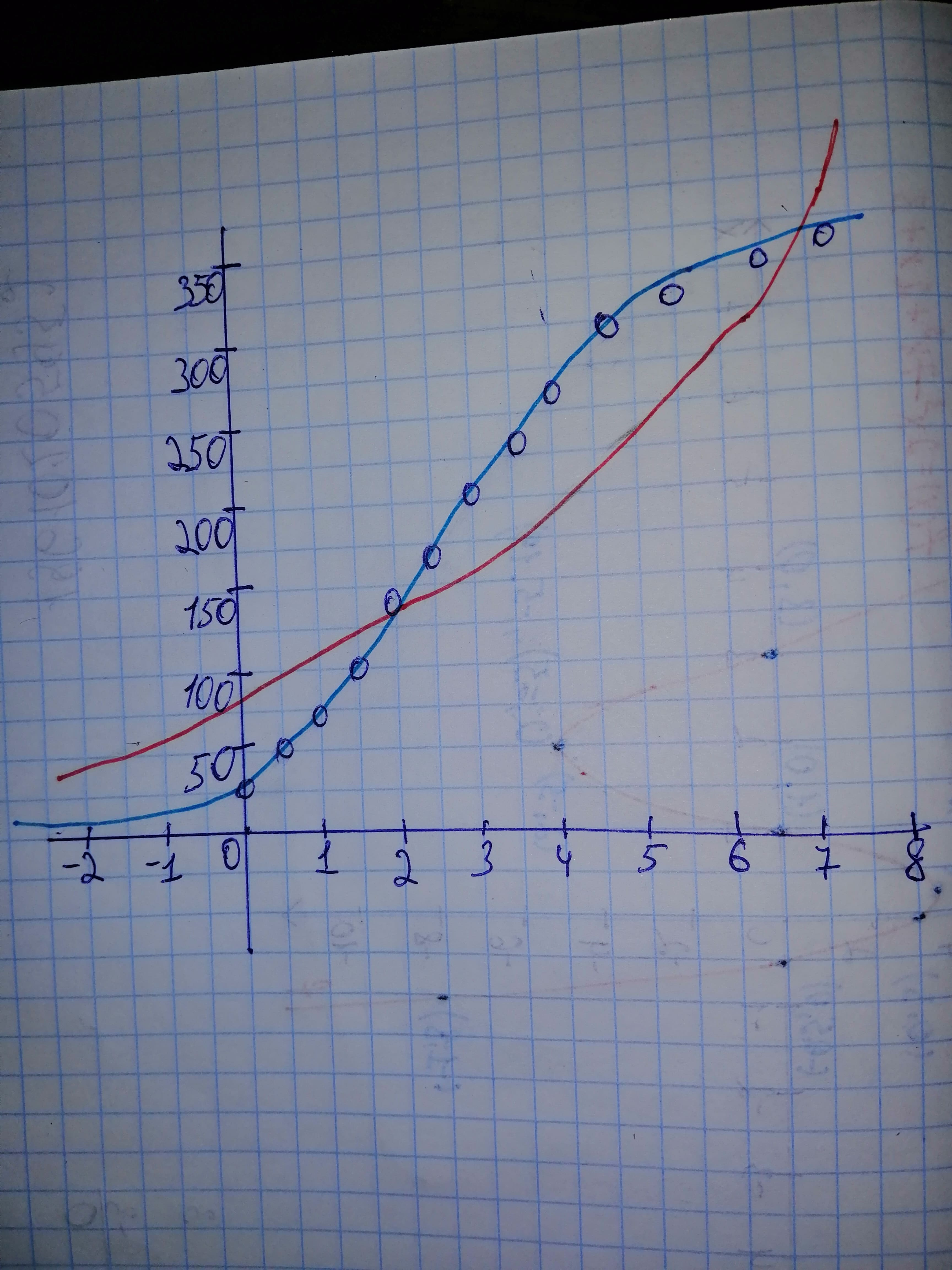# The table gives the number of active Twitter users worldwide, semiannually from 2010 to 2016.glamrockqueen7 2021-02-09 Answered

The table gives the number of active Twitter users worldwide, semiannually from 2010 to 2016. $\begin{array}{|cccc|}\hline \text{Years since}& \text{January 1, 2010}& \text{Twitter user}& \text{(millions)}\\ 0& 30& 3.5& 232\\ 0.5& 49& 4.0& 255\\ 1.0& 68& 4.5& 284\\ 1.5& 101& 5.0& 302\\ 2.0& 138& 5.5& 307\\ 2.5& 167& 6.0& 310\\ 3.0& 204& 6.5& 317\\ \hline\end{array}$

Use a calculator or computer to fit both an exponential function and a logistic function to these data. Graph the data points and both functions, and comment on the accuracy of the models.

You can still ask an expert for help

• Questions are typically answered in as fast as 30 minutes

Solve your problem for the price of one coffee

• Math expert for every subject
• Pay only if we can solve itVelsenw

Step 1 Using Desmos, first add a table. (click on "+" at the upper left) and add the numbers. It should look something like in this image:

$\begin{array}{|cc|}\hline {x}_{1}& {y}_{1}\\ 0& 30\\ 0.5& 49\\ 1& 68\\ 1.5& 101\\ 2& 138\\ 2.5& 167\\ 3& 204\\ 3.5& 232\\ 4& 255\\ 4.5& 284\\ 5& 302\\ 5.5& 307\\ 6& 310\\ 6.5& 317\\ \hline\end{array}$

Step 2 Next, you can get the exponential model form by typing in the next box below the one with the table: Using the output from Desmos, we can write the exponential model as follows:

${R}^{2}=0.8627$ ${e}_{1}$
$a=89.1544$
$b=1.24572$

Step 3 You can get the logistic model form by typing: $\text{Undefined control sequence \rightarrowM}$ Using the output from Desmos, we can write the logistic model as follows:

${R}^{2}=0.9988$
${e}_{1}$
$m=325.764$
$A=8.75376$
$k=0.892708$

Step 4 The logistic model is more accurate because it has larger ${R}^{2}$ value. You can also see this from the graph below: The exponential and the logistic models are represented by the red and blue color curves respectively.